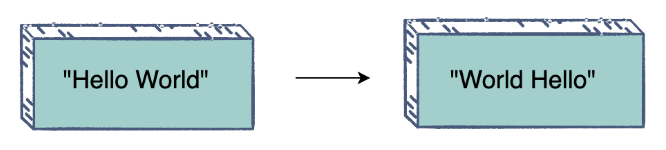# Reverse Words in a Sentence

## Problem Statement

Reverse the order of words in a given sentence (an array of characters). Take the “Hello World” string for example:## Hint

• Note how the words are separated by whitespaces

## Try it yourself

void reverse_words(char * sentence) {  //TODO: Write - Your - Code}

## Solution

void str_rev(char * str, int len) {  if (str == nullptr || len < 2) {    return;  }  char * start = str;  char * end = str + len - 1;  while (start < end) {    if (start != nullptr && end != nullptr) {      char temp = * start;      * start = * end;      * end = temp;    }    start++;    end--;  }}void reverse_words(char * sentence) {  // Here sentence is a null-terminated string ending with char '\0'.  if (sentence == nullptr) {    return;  }  // To reverse all words in the string, we will first reverse  // the string. Now all the words are in the desired location, but  // in reverse order: "Hello World" -> "dlroW olleH".  int len = strlen(sentence);  str_rev(sentence, len);  // Now, let's iterate the sentence and reverse each word in place.  // "dlroW olleH" -> "World Hello"  char * start = sentence;  char * end;  while (true) {    // find the  start index of a word while skipping spaces.    while (start && * start == ' ') {      ++start;    }    if (start == nullptr || * start == '\0') {      break;    }    // find the end index of the word.    end = start + 1;    while (end && * end != '\0' && * end != ' ') {      ++end;    }    // let's reverse the word in-place.    if (end != nullptr) {      str_rev(start, (end - start));    }    start = end;  }}int main() {  string str = "Hello World!";  char* a = const_cast<char*>(str.c_str());  cout << a << endl;  reverse_words(a);  cout << a << endl;}

## Solution Explanation

### Runtime complexity

The runtime complexity of this solution is linear, O(n).

Position of all the elements in the sentence is changed.

### Memory complexity

The memory complexity of this solution is constant, O(1).

### Solution Breakdown

Here is how the solution works:

• Reverse the string.
• Traverse the string and reverse each word in place.

Practice problems like this and many more by checking out our Grokking the Coding Interview: Patterns for Coding Questions course!

Learn in-demand tech skills in half the time